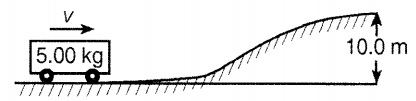# Quiz on Enertia, Kinetic Energy, and Coulomb's Law

Question 1: An object that is negatively charged:
• has lost electrons.
• has gained electrons.
• has zero energy.
• has more electrons than protons.
Question 2: Describe the motion of a driver not wearing his/her seat-belt when the car suddenly stops.

• The person and car will stop together.
• The car is still in motion.
• The driver does not move.
• The car will stop and the person will keep moving forward because of inertia.
Question 3: If visitors travel all the way to the moon. Describe the change.

• mass of the visitors.
• weight of the visitors.
• energy of the visitors..
• height of the visitors.
Question 4: The diagram below shows a moving, 5-kg cart at the foot of a hill 10.0 meters high.For the cart to reach the top of the hill, what is the minimum kinetic energy of the cart in the position shown?
Assume that energy loss due to friction is negligable

• 49 J
• 490 J
• 4.9 J
• 0 J
Question 5: See the figure below and determine the kinetic energy of the rock when it reaches the ground.• 49,000 J
• 45,000 J
• 44,100 J
• 42,000 J
Question 6: A ball moving at 80 m/s has a momentum of 20 kg·m/s.
Calculate the mass of the ball.

• 0.2 kg.
• 0.25 kg.
• 0.35 kg.
• 0.5 kg.
Question 7: Consider a truck has a linear momentum of 30,000 kg⋅m/s, and it is moving at 10 m/s.
Calculate is its mass.

• 2,000 kg.
• 3,000 kg.
• 4,000 kg.
• 5,000 kg.
Question 8: A squirrel leaps from its resting position at the near tree onto a golf course.
If the squirrel has a mass of 0.8 kg and the acceleration of the leap is 5 m/s2,
Find the force the squirrel exerts when leaping?

• 5 m/s2
• 4 m/s2
• 3 m/s2.
• 2 m/s2
Question 9: How do you compare magnitude of the electrical charge carried by an electron with he magnitude of the charge on an electron proton?

• No comparison.
• Exactly same.
• Electron's charge is bigger
• Proton's charge is bigger
Question 10: The magnitude of the electric force between two protons is 5× 10− 26 N.
Find the distance between the two protons.

• 0.1 m
• 0.01 m
• 0.005 m
• 0.071 m
Question 11: A sister pushes her 10-kg little brother, who is sitting on a swing.
Assumne the sister exerts a 8-newton force on the child for 0.40 second, calculate the magnitude of the impulse imparted to the child by his sister.

• 0.3 N
• 0.4 N
• 0.5 N
• 0.2 N
Question 12: Which of the following is an energy unit?

• Ohm
• Ampere
• Volt
• Watt
• Joule